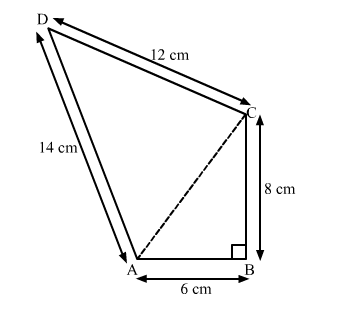# The sides of a quadrilateral ABCD taken in order are 6 cm, 8 cm,

Question:

The sides of a quadrilateral $A B C D$ taken in order are $6 \mathrm{~cm}, 8 \mathrm{~cm}, 12 \mathrm{~cm}$ and $14 \mathrm{~cm}$ respectively and the angle between the first two sides is a right angle. Find its area. (Given, $\sqrt{6}=2.45$ ).

Solution:

In the given figure, ABCD is a quadrilateral with sides of length 6 cm, 8 cm, 12 cm and 14 cm respectively and the angle between the first two sides is a right angle.Join AC.

In right angled ∆ABC,

$A C^{2}=A B^{2}+B C^{2}$           (Pythagoras Theorem)

$\Rightarrow A C^{2}=6^{2}+8^{2}$

$\Rightarrow A C^{2}=36+64$

$\Rightarrow A C^{2}=100$

⇒ AC = 10 cm

Area of $\triangle A B C=\frac{1}{2} \times A B \times B C$

$=\frac{1}{2} \times 6 \times 8$

$=24 \mathrm{~cm}^{2}$             ....(1)

In ∆ACD,
The sides of the triangle are of length 10 cm, 12 cm and 14 cm.
∴ Semi-perimeter of the triangle is

$s=\frac{10+12+14}{2}=\frac{36}{2}=18 \mathrm{~cm}$

∴ By Heron's formula,

Area of $\Delta A C D=\sqrt{s(s-a)(s-b)(s-c)}$

$=\sqrt{18(18-10)(18-12)(18-14)}$

$=\sqrt{18(8)(6)(4)}$

$=24 \sqrt{6} \mathrm{~cm}^{2}$

$=24(2.45) \mathrm{cm}^{2}$

$=58.8 \mathrm{~cm}^{2}$        $\ldots(2)$

Thus,
Area of quadrilateral ABCD = Area of ∆ABC + Area of ∆ACD

$=(24+58.8) \mathrm{cm}^{2}$

$=82.8 \mathrm{~cm}^{2}$

Hence, the area of quadrilateral $A B C D$ is $82.8 \mathrm{~cm}^{2}$.